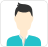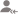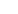true# Measures of Central Tendency Updates

Post a Lesson

Answered on 02/01/2018 CBSE/Class 10/Mathematics Tuition/Class IX-X Tuition Measures of Central TendencyHighly Qualified Tutor

3 mean - 2 median
Dislike Bookmark

Answered on 26/11/2016 CBSE/Class 10/Mathematics Tuition/Class IX-X Tuition Measures of Central Tendency

Find the mean of first 15 natural numbers?Ashwini

commerce

9
Dislike Bookmark

Answered on 27 Aug CBSE/Class 10/Mathematics Tuition/Class IX-X Tuition Measures of Central Tendency

The following data have been arranged in ascending order of magnitudes: 59, 62, 65, x, x + 2, 72, 85,... read more
The following data have been arranged in ascending order of magnitudes: 59, 62, 65, x, x + 2, 72, 85, 94. If the median of the data is 69. Find the value of x? read lessVenkatesan Iyer

Tutor

No of observations = 8 (even) 8/2=4 Median =(4th data + 5th data)/2 69= (x+(x+2))/2 69*2=2x+2 138-2=2x 136/2=x 68=x
Dislike Bookmark

Answered on 29/11/2016 CBSE/Class 10/Mathematics Tuition/Class IX-X Tuition Measures of Central Tendency

Find the mode of the following data: 14, 25, 14, 28, 18, 17, 18, 14, 23, 22, 14, 18?Mamta Mund

Maths Tutor

14 has highest frequency i.e., 4. So, mode is 14.
Dislike Bookmark

Answered on 05/12/2016 CBSE/Class 10/Mathematics Tuition/Class IX-X Tuition Measures of Central Tendency

If the mean of 10, 12, 18, 13, x, 17 is 15. Find the value of x?Chandan Singh

Tutor

Mean = Sum of total numbers/ total number of numbers 10+12+18+13+x+17/6=15 70+x=90 Hence, x=90-70=20 Ans.
Dislike Bookmark

Answered on 29/11/2016 CBSE/Class 10/Mathematics Tuition/Class IX-X Tuition Measures of Central Tendency

The mean of 13 items is 14. If the mean of first 7 items is 12 and that of the last 7 items is 16. Find the 7th item?Tapas Bhattacharya

Tutor

Sum of 13 items=S = 13 x 14=182. Sum of 1st 7 items=S1 = 7 x 12=84. Sum of last 7 items=S2 = 7 x 16=112. So, S1 + S2=Sum of 13 items + the 7th item=S + 7th item --> 182 + 7th item=84 + 112=196. --> 7th item = 196 - 182 = 14 Answer: 14.
Dislike Bookmark

Answered on 27/11/2016 CBSE/Class 10/Mathematics Tuition/Class IX-X Tuition Measures of Central Tendency

For what value of x, the mode of the following data is 10: 7, 6, 10, x, 7, 5, 12, 14?Mamta Mund

Maths Tutor

10
Dislike Bookmark

Answered on 29/11/2016 CBSE/Class 10/Mathematics Tuition/Class IX-X Tuition Measures of Central Tendency

Find the median of the following data 31, 15, 19, 12, 30, 45, 60, 50, 25, 40. If 40 is replaced by 20,... read more
Find the median of the following data 31, 15, 19, 12, 30, 45, 60, 50, 25, 40. If 40 is replaced by 20, what will be the new median? read lessComplete Package

Arranging the data in ascending order, we get: 12, 15, 19, 25, 30, 31, 40, 45, 50, 60. As there are 10 values and 10 is an even number, the mean of 5th and 6th value will be the median. So, median=(30+31)/2 = 30.5 If 40 is replaced by 20, we get: 12, 15, 19, 20, 25, 30, 31, 45, 50, 60. So, median will... read more
Arranging the data in ascending order, we get: 12, 15, 19, 25, 30, 31, 40, 45, 50, 60. As there are 10 values and 10 is an even number, the mean of 5th and 6th value will be the median. So, median=(30+31)/2 = 30.5 If 40 is replaced by 20, we get: 12, 15, 19, 20, 25, 30, 31, 45, 50, 60. So, median will be (25+30)/2=27.5. read less
Dislike Bookmark

Answered on 05/12/2016 CBSE/Class 10/Mathematics Tuition/Class IX-X Tuition Measures of Central Tendency

What is the average of the data: 4, 5, 6, 8, 10, 11, 12?Chandan Singh

Tutor

Average= (Sum of total data)/no. of data = (4+5+68+10+11+12)/7= 8 (Ans).
Dislike Bookmark

Answered on 25/11/2016 CBSE/Class 10/Mathematics Tuition/Class IX-X Tuition Measures of Central Tendency

What is the mean of first eight prime numbers?Saraswathi S.

Tutor

2, 3, 5, 7, 11, 13, 17, 19 are the first 8 prime numbers.
Dislike Bookmark

UrbanPro.com helps you to connect with the best in India. Post Your Requirement today and get connected.

Overview

Questions 25

Total Shares30 Followers

## Top Contributors

Connect with Expert Tutors & Institutes for Measures of Central Tendencyx